## Time and Work For SBI PO Set – 01

1) Certain men completed a piece of work in 20 days. One man starts the work and the after every day one more man joins the work and this continues till the work is completed. The work was completed in 24 days. Then how many men completed the work in 20 days?

a) 25

b) 20

c) 30

d) 10

e) 15

b) 20

2. A and B are two workers. When A completes one-third of the work and hands over to B, it takes B 40 more days to complete the work. When B completes one-third of the work and hands over to A, it takes A 24 more days to finish the work. How many days would it take to finish the work if both A and B start working together?

a) 10 days

b) 22.5 days

c) 30 days

d) 40 days

e) Cannot be determined

b) 22.5 days

3. Akshaya can write a book in 3 days less time than her brother. Initially Akshaya writes it for 4 days and then her brother takes over remaining work and completes it. If altogether 14 days were required to write a book, then in how many days would each of them take alone to finish it?

a) 14 days, 17 days

b) 5 days, 18 days

c) 13 days, 16 days

d) 12 days, 15 days

e) None of these

d) 12 days, 15 days

4. A can do a certain work in the same time in which B and C together can do it. If A and B together could do it in 10 days and C alone in 50 days, then B alone could do it in?

a) 15 days

b) 20 days

c) 25 days

d) 30 days

e) None of these

c) 25 days

Explanation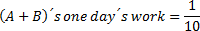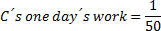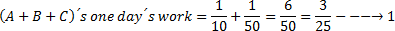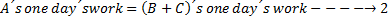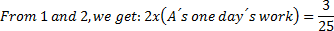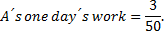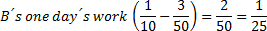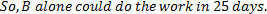5. 3 men and 4 women can complete a piece of work in 12 while the same is finished by 2 men and one woman in 15 days. A boy who is 25% less efficient than that of man can complete the piece of work in how many days.

a) 400/11

b) 420/11

c) 430/11

d) 450/11

e) None of these

a) 400/11

Explanation

3m + 4w = 1/12

2m + w = 1/15. After solving we one day work of man = 33/900

One day work of the boy is= 75/100*(33/900) Â = 11/400. So no of days needed will be 400/11.

6) Arun can do a piece of work in 30 days. Rohan who is 40% more efficient than arun can finish the work in how many days.

a) 152/7

b)  150/7

c) 145/7

d) 142/7

e)None of these

b)  150/7

Explanation

In one day work done by arun is 1/30 and rohan is 40% more efficient than arun , so one day work of rohan = (140/100) *1/30 = 7/150.

So time taken by rohan will be 150/7

7. Three printing press P, Q and R can print 1 lack books in 5 hrs, 15 hrs and 10 hrs respectively. If all the press starts at 10 am and press R is stopped after 2 hrs then at what time work will be complete.

a) 12 pm

b) 1 pm

c) 2pm

d) 3pm

e) None of these

b) 1 pm

Explanation

Time taken by P = 5hrs, Q = 15hr and R = 10 hrs

(1/5+1/15+1/10) *2 + (1/5 + 1/15) *X = 1 (X = time taken to do remaining work)

after solving we get X = 1. So 2+1 = 3hrs. i.e at 1pm work will be completed.

8) A total of 960 rupees is given to three people A, B and C.Â  A and B together can complete 7/12 and C and B together can complete 5/12. So, how much amount should be given to A.

a) 360

b) 460

c) 280

d) 660

e) None of these

c) 280

Explanation

(work done by) : (work done by B and C)

A:B = 7:12 & C:B = 5:12 => A:B:C = 7:12:5

So amount to be paid to A = (7/24) *960 = 280

9. If P can do 1/3 of the work in 5 days and Q can do Â¼ of the work in 6 days, then how much money will Q get if theyÂ  were paid a total of 390 rupee.

a) 120

b) 150

c) 170

d) 190

e) None of these

b) 150

Explanation

can alone complete the wholeÂ  work in 15 days and Q can complete the same work alone in 24 days. So ratio of work done by them 1/15 : 1/24 i.e 8 : 5

Q get = (5/13) *390 = 150

10. Siva can complete the job in 12hrs. Aswin can complete the job in 8 hrs. If Siva worked for 6hrs then quit. How many hours will Aswin alone take to complete the remaining work ?

a) 4hrs

b) b3hrs

c) 5hrs

d) 7hrs

e) None of these

a) 4hrs

Explanation

6/12+x/8= 1

12+3x = 24

3x = 12

X =4